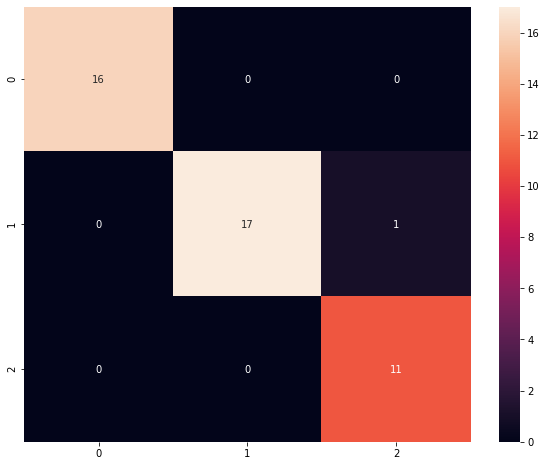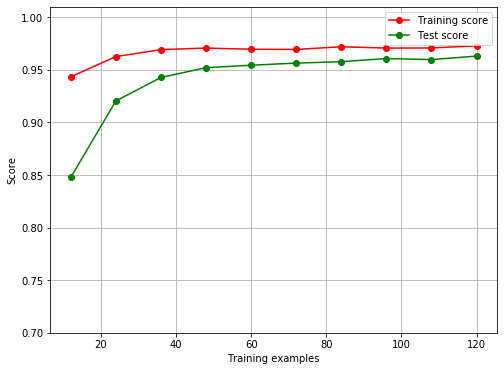# Hands-On: Machine Learning Methods¶

### AI and Predictive Analytics in Data-Center Environments - http://dcai.bsc.es¶

On this hands-on we will take a first look to how to create, train and evaluate a Machine Learning model with Python and Scikit-learn (abbreviated Sklearn). Sklearn is a free software machine learning library for the Python programming language featuring various classification, regression and clustering algorithms, and it is widely used both in industry and academia.

For this first example we will use the Iris dataset that has been already introduced in the course. Sklearn conveniently includes a pre-processed version of the Iris dataset in its datasets package, saving us the hassle of downloading and wrangling with the data. We will start by importing the package sklearn.datasets, which contains the Iris data:

In :
from sklearn import datasets


Now we can load the Iris data using the method load_iris:

In :
iris_ds = datasets.load_iris()


To avoid having to type iris_ds. to access the features and labels of the dataset we will save the dataset's features (iris_ds.data) and labels (iris_ds.target) into variables called X and y respectively:

In :
X = iris_ds.data
y = iris_ds.target


## Training and validation datasets¶

A crucial step in the model creation process is being able to test the model in some data that has not been used to train it. Because we only have one dataset and we are not able to get more data on the Iris flowers, we will need to save some of the data we have in order to use it for validation purposes. We can do this using Sklearn's train_test_split, which will split the dataset in two with a given train-test dataset size ratio:

In :
from sklearn.model_selection import train_test_split
X_train, X_test, y_train, y_test = train_test_split(X, y, test_size=0.3, random_state=0)


You can see that we have also passed a random_state parameter to train_test_split. random_state sets a seed for the Random Number Generator (RNG) used to perform the split, and it allows us to obtain the same results at every run of this example to ensure repeatability of the results.

## Building the classification model¶

Once our training and validation datasets ready we can move on to creating our model. For this first classification example we will use a Logistic Regression model:

In :
from sklearn.linear_model import LogisticRegression
log_reg = LogisticRegression( solver='lbfgs', multi_class='auto', random_state=0 )


Again, we have passed a random_state. You will see this quite often when working with Sklearn. We have also passed two other parameters, solver and multi_class. They are actually passing the default values, but we need to do so to silence some warnings (solved for future versions of Sklearn).

Right now our logistic regression model is like an empty box, as we have not trained it with any data. Sklearns uses a common API for all its models, with fit used to train the model on training data and predict used to make predictions on data. We can then train the model using fit:

In :
log_reg.fit( X_train, y_train );


## Evaluating the model¶

Now that the model has been trained one of the scores of the quality of the fit we can obtain is the R^2 score, which indicates how much of the variance of the training data is explained by the model:

In :
train_score = log_reg.score( X_train, y_train )
print("R2 Score: {}".format(train_score))

R2 Score: 0.9809523809523809


That's a fairly good R2 score! According to it our model can explain 98% of the variabnce of the training data. The Iris dataset is a quite simple dataset, so this result should not be surprising.

Let's now try and make predictions on the validation data using predict. We can calculate the Mean Squared Error of the prediction versus the real labels with mean_squared_error:

In :
lr_test_prediction = log_reg.predict(X_test)


## Confusion Matrix¶

We can generate the prediction's confusion matrix with confusion_matrix:

In :
from sklearn.metrics import confusion_matrix
print("Logistic Regression confusion matrix:\n{}".format(confusion_matrix(y_test, lr_test_prediction)))

Logistic Regression confusion matrix:
[[16  0  0]
[ 0 17  1]
[ 0  0 11]]


Although the confusion matrix above has all the information we need it's format is not very appealing. We can generate a better visualization using a heatmap from the Seaborn package:

In :
import matplotlib.pyplot as plt
import seaborn as sns
plt.figure(figsize = (10,8))
sns.heatmap(confusion_matrix(y_test, lr_test_prediction), annot=True);## Visualizing error¶

To be able to visualize how the test and training errors evolve as the training process progresses it would be interesting to plot them with regards to the numbers of samples used in training. This can be achieved with the learning_curve method:

In :
# Courtesy of Sklearn documentation
import numpy as np
from sklearn.model_selection import learning_curve, ShuffleSplit

# Cross validation with 100 iterations to get smoother mean test and train
# score curves, each time with 20% data randomly selected as a validation set.
cv = ShuffleSplit(n_splits=100, test_size=0.2, random_state=0)

estimator = LogisticRegression(solver='lbfgs', multi_class='auto', random_state=0)

plt.figure(figsize=(8,6))
plt.ylim(0.7, 1.01)
plt.xlabel("Training examples")
plt.ylabel("Score")

train_sizes, train_scores, test_scores = learning_curve( estimator, X, y, cv=cv, n_jobs=-1, train_sizes=np.linspace(.1, 1.0, 10) )
plt.grid()

plt.plot( train_sizes, np.mean(train_scores, axis=1), 'o-', color="r", label="Training score" )
plt.plot( train_sizes, np.mean(test_scores, axis=1), 'o-', color="g", label="Test score" )
plt.legend( loc="best" );